# Global behavior for a diffusive predator-prey system with Holling type II functional response

## Abstract

A strongly coupled self- and cross-diffusion predator-prey system with Holling type II functional response is considered. Using the energy estimate, Sobolev embedding theorem and bootstrap arguments, the global existence of non-negative classical solutions to this system in which the space dimension is not more than five is obtained.

MSC: 35K50, 35K55, 35K57, 92D40.

## 1 Introduction

In this paper, we consider the global existence of non-negative classical solutions to the following diffusion predator-prey system with Holling type II functional response:

$\left\{\begin{array}{c}{u}_{t}=\mathrm{\Delta }\left({d}_{1}u+{\alpha }_{11}{u}^{2}\right)+\alpha u\left(1-\frac{u}{K}\right)-\frac{\beta muv}{1+amu},\phantom{\rule{1em}{0ex}}x\in \mathrm{\Omega },t>0,\hfill \\ {v}_{t}=\mathrm{\Delta }\left({d}_{2}v+{\alpha }_{21}uv+{\alpha }_{22}{v}^{2}\right)-rv+\frac{c\beta muv}{1+amu},\phantom{\rule{1em}{0ex}}x\in \mathrm{\Omega },t>0,\hfill \\ {\partial }_{\eta }u\left(x,t\right)={\partial }_{\eta }v\left(x,t\right)=0,\phantom{\rule{1em}{0ex}}x\in \partial \mathrm{\Omega },t>0,\hfill \\ u\left(x,0\right)={u}_{0}\left(x\right)\ge 0,v\left(x,0\right)={v}_{0}\left(x\right)\ge 0,\phantom{\rule{1em}{0ex}}x\in \mathrm{\Omega },\hfill \end{array}$
(1.1)

where Ω is a bounded region in ${\mathbb{R}}^{n}$ ($n\ge 1$) with a smooth boundary Ω; η is the outward normal on Ω, ${\partial }_{\eta }=\partial /\partial \eta$; ${u}_{0}\left(x\right)$ and ${v}_{0}\left(x\right)$ are non-negative smooth functions and are not identically zero; u and v denote the population densities of predator and prey, respectively; α, β, r, a, K, m and c are positive constants, and $m\in \left(0,1\right]$; ${d}_{1}$ and ${d}_{2}$ are the diffusion rates of the two species; ${\alpha }_{ij}$ ($i,j=1,2$) are given non-negative constants, ${\alpha }_{11}$ and ${\alpha }_{22}$ are self-diffusion rates; ${\alpha }_{21}$ is the cross-diffusion rate. It means that the diffusion is from one species of high-density areas to the other species of low-density areas. See [1, 2] for more details on the ecological backgrounds of this system.

Obviously, the non-negative equilibrium solutions of system (1.1) are $\left(K,0\right)$ and $\left({u}_{\ast },{v}_{\ast }\right)=\left(\frac{r}{m\left(c\beta -ar\right)},\frac{\alpha cKm\left(c\beta -ar\right)-\alpha cr}{K{m}^{2}{\left(c\beta -ar\right)}^{2}}\right)$. For the reaction-diffusion problem of system (1.1), i.e., ${\alpha }_{ii}=0$ ($i=1,2$), the global attraction, persistence and stability of non-negative equilibrium solutions are studied in . The main result can be summarized as follows:

1. (1)

If $m<\frac{r}{K\left(c\beta -ar\right)}$, a semi-trivial solution $\left(K,0\right)$ is globally asymptotically stable;

2. (2)

If $\frac{r}{K\left(c\beta -ar\right)} and $c\beta >ar$, a unique positive constant solution $\left({u}_{\ast },{v}_{\ast }\right)$ is globally asymptotically stable;

3. (3)

If $\frac{r}{K\left(c\beta -ar\right)} and $c\beta >ar$, a positive constant solution$\left({u}_{\ast },{v}_{\ast }\right)$ is locally asymptotically stable.

In view of the study of dynamic behavior of a predator-prey reaction-diffusion system with Holling type II functional response, a natural problem is what the global behavior for a predator-prey cross-diffusion system (1.1) is. To the best of our knowledge, the existing results are very few. In this paper, we consider the space dimension to be less than six, and initial function ${u}_{0}\left(x\right)$ and ${v}_{0}\left(x\right)$ under some smooth conditions, using the energy estimate, Sobolev embedding theorem and bootstrap arguments, we consider the global existence of non-negative classical solutions for system (1.1).

We denote ${Q}_{T}=\mathrm{\Omega }×\left(0,T\right)$. $u\in {W}_{q}^{2,1}\left({Q}_{T}\right)$ means that u, ${u}_{{x}_{i}}$, ${u}_{{x}_{i}{x}_{j}}$ ($i,j=1,\dots ,n$) and ${u}_{t}$ are in ${L}^{q}\left({Q}_{T}\right)$. ${\parallel u\parallel }_{{L}^{p,q}\left({Q}_{T}\right)}={\left[{\int }_{0}^{T}{\left({\int }_{\mathrm{\Omega }}{|u\left(x,t\right)|}^{p}\phantom{\rule{0.2em}{0ex}}\mathrm{d}x\right)}^{\frac{q}{p}}\phantom{\rule{0.2em}{0ex}}\mathrm{d}t\right]}^{\frac{1}{q}}$. ${\parallel u\parallel }_{{V}_{2}\left({Q}_{T}\right)}={sup}_{0\le t\le T}{\parallel u\left(\cdot ,t\right)\parallel }_{{L}^{2}\left(\mathrm{\Omega }\right)}+{\parallel \mathrm{\nabla }u\parallel }_{{L}^{2}\left({Q}_{T}\right)}$.

## 2 Auxiliary results

Lemma 2.1 Let $\left(u,v\right)$ be the solution of (1.1). There exists a positive constant ${M}_{0}\left(\ge 1\right)$ such that

$0\le u\le {M}_{0},\phantom{\rule{2em}{0ex}}0\le v,\phantom{\rule{1em}{0ex}}\mathrm{\forall }t\ge 0.$
(2.1)

Proof Firstly, the existence of local solutions for system (1.1) is given in . Roughly speaking, if ${u}_{0},{v}_{0}\in {W}_{p}^{1}\left(\mathrm{\Omega }\right)$, $p>1$, there exists the maximum $T\le +\mathrm{\infty }$ such that system (1.1) admits a unique non-negative solution

$u,v\in C\left(\left[0,T\right),{W}_{p}^{1}\left(\mathrm{\Omega }\right)\right)\cap {C}^{\mathrm{\infty }}\left(\left(0,T\right),{C}^{\mathrm{\infty }}\left(\mathrm{\Omega }\right)\right).$
(2.2)

If

$sup\left\{{\parallel u\left(\cdot ,t\right)\parallel }_{{w}_{p}^{1}\left(\mathrm{\Omega }\right)},{\parallel v\left(\cdot ,t\right)\parallel }_{{w}_{p}^{1}\left(\mathrm{\Omega }\right)}:0

then $T=+\mathrm{\infty }$.

Choose ${M}_{0}=max\left\{K,{\parallel {u}_{0}\parallel }_{{L}^{\mathrm{\infty }}\left(\mathrm{\Omega }\right)}\right\}$. By use of the maximum principle, the non-negative solution of system (1.1) can be derived from the maximum principle, i.e., $u,v\ge 0$ for all $t\ge 0$. This completes the proof of Lemma 2.1. □

Lemma 2.2 Let $X=\left({d}_{1}+{\alpha }_{11}u\right)u$, $u\in {L}^{\mathrm{\infty }}\left({Q}_{T}\right)$ for the solution to the following equation:

$\begin{array}{c}{u}_{t}=\mathrm{\Delta }\left[\left({d}_{1}+{\alpha }_{11}u\right)u\right]+\alpha u\left(1-\frac{u}{K}\right)-\frac{\beta muv}{1+amu},\phantom{\rule{1em}{0ex}}\left(x,t\right)\in \mathrm{\Omega }×\left(0,T\right),\hfill \\ {\partial }_{\eta }u=0,\phantom{\rule{1em}{0ex}}\left(x,t\right)\in \partial \mathrm{\Omega }×\left(0,T\right),\hfill \\ u\left(x,0\right)={u}_{0}\left(x\right)\ge 0,\phantom{\rule{1em}{0ex}}x\in \mathrm{\Omega },\hfill \end{array}$

where ${d}_{1}$, ${\alpha }_{11}$ are positive constants and $0\le u\in {L}^{2}\left({Q}_{T}\right)$. Then there exists a positive constant $C\left(T\right)$, depending on ${\parallel {u}_{0}\parallel }_{{W}_{2}^{1}\left(\mathrm{\Omega }\right)}$ and ${\parallel {u}_{0}\parallel }_{{L}^{\mathrm{\infty }}\left(\mathrm{\Omega }\right)}$, such that

${\parallel X\parallel }_{{W}_{2}^{2,1}\left({Q}_{T}\right)}\le C\left(T\right).$
(2.3)

Furthermore,

$\mathrm{\nabla }X\in {V}_{2}\left({Q}_{T}\right),\phantom{\rule{2em}{0ex}}\mathrm{\nabla }u\in {L}^{\frac{2\left(n+2\right)}{n}}\left({Q}_{T}\right).$
(2.4)

Proof From $X=\left({d}_{1}+{\alpha }_{11}u\right)u$, it is easy to find that

${X}_{t}=\left({d}_{1}+2{\alpha }_{11}u\right)\mathrm{\Delta }X+{C}_{1}-{C}_{2}v,$
(2.5)

where ${C}_{1}={d}_{1}\alpha u+\left(2{\alpha }_{11}\alpha -\frac{{d}_{1}\alpha }{K}\right){u}^{2}-\frac{2{\alpha }_{11}\alpha }{K}{u}^{3}$ and ${C}_{2}=\frac{\beta mu}{1+amu}\left({d}_{1}+2{\alpha }_{11}u\right)$. ${C}_{1}$ and ${C}_{2}$ are bounded in ${Q}_{T}$ from (2.1). Multiplying (2.5) by $-\mathrm{\Delta }X$ and integrating by parts over ${Q}_{t}$ yields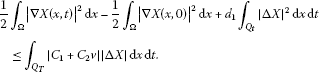(2.6)

Using the Hölder inequality and Young inequality to estimate the right-hand side of (2.6), we have

$\begin{array}{rcl}{\parallel {C}_{1}+{C}_{2}v\parallel }_{{L}^{2}\left({Q}_{T}\right)}{\parallel \mathrm{\Delta }X\parallel }_{{L}^{2}\left({Q}_{T}\right)}& \le & {m}_{1}\left(1+{\parallel v\parallel }_{{L}^{2}\left({Q}_{T}\right)}\right){\parallel \mathrm{\Delta }X\parallel }_{{L}^{2}\left({Q}_{T}\right)}\\ \le & \frac{{m}_{1}^{2}{\left(1+{M}_{3}\right)}^{2}}{2{d}_{1}}+\frac{{d}_{1}}{2}{\parallel \mathrm{\Delta }X\parallel }_{{L}^{2}\left({Q}_{T}\right)}^{2}\end{array}$
(2.7)

with some ${m}_{1}>0$. Substituting (2.7) into (2.6), we obtain

$\underset{0\le t\le T}{sup}{\int }_{\mathrm{\Omega }}{|\mathrm{\nabla }X\left(x,t\right)|}^{2}\phantom{\rule{0.2em}{0ex}}\mathrm{d}x+{d}_{1}{\int }_{{Q}_{t}}{|\mathrm{\Delta }X|}^{2}\phantom{\rule{0.2em}{0ex}}\mathrm{d}x\phantom{\rule{0.2em}{0ex}}\mathrm{d}t\le {m}_{2},$

where ${m}_{2}$ depends on ${\parallel {u}_{0}\parallel }_{{W}_{2}^{1}\left(\mathrm{\Omega }\right)}$ and ${\parallel {u}_{0}\parallel }_{{L}^{\mathrm{\infty }}\left(\mathrm{\Omega }\right)}$. So, we know $\mathrm{\nabla }X\in {V}_{2}\left({Q}_{T}\right)$. Since $X\in {L}^{2}\left({Q}_{T}\right)$, it follows from the elliptic regularity estimate [, Lemma 2.3] that

${\int }_{{Q}_{T}}{|{X}_{{x}_{i}{x}_{j}}|}^{2}\phantom{\rule{0.2em}{0ex}}\mathrm{d}x\phantom{\rule{0.2em}{0ex}}\mathrm{d}t\le {m}_{3},\phantom{\rule{1em}{0ex}}i,j=1,\dots ,n.$

From (2.5), we have ${X}_{t}\in {L}^{2}\left({Q}_{T}\right)$. Hence, ${\parallel X\parallel }_{{W}_{2}^{2,1}\left({Q}_{T}\right)}\le C\left(T\right)$. Moreover, (2.4) can be obtained by use of the Sobolev embedding theorem. □

Lemma 2.3 Assume that $w\in {W}_{P}^{2,1}\left({Q}_{T}\right)\cap {C}^{2,1}\left(\overline{\mathrm{\Omega }}×\left[0,T\right)\right)$ is a bounded function satisfying

with the boundary condition $\frac{\partial w}{\partial v}\le 0$ on ${\partial }_{{Q}_{T}}$, where $f\in {L}^{P}\left({Q}_{T}\right)$. Then W is in ${L}^{2p}\left({Q}_{T}\right)$.

The proof of the above lemma can be found in [, Proposition 2.1].

The following result can be derived from Lemma 2.3 and Lemma 2.4 of .

Lemma 2.4 Let $p>1$, $\stackrel{˜}{p}=2+\frac{4p}{n\left(p+1\right)}$. If

$\underset{0\le t\le T}{sup}{\parallel w\parallel }_{{L}^{\frac{2p}{p+1}}\left(\mathrm{\Omega }\right)}+{\parallel \mathrm{\nabla }w\parallel }_{{L}^{2}\left({Q}_{T}\right)}<\mathrm{\infty },$

and there exist positive constants $\beta \in \left(0,1\right)$ and ${C}_{T}$ such that ${\int }_{\mathrm{\Omega }}{|w\left(\cdot ,t\right)|}^{\beta }\phantom{\rule{0.2em}{0ex}}\mathrm{d}x\le {C}_{T}$ ($\mathrm{\forall }t\le T$), there exists a positive constant ${M}^{\prime }$, independent of w but possibly depending on n, Ω, p, β and ${C}_{T}$, such that

${\parallel w\parallel }_{{L}^{\stackrel{˜}{p}}\left({Q}_{T}\right)}\le {M}^{\prime }\left\{1+{\left(\underset{0\le t\le T}{sup}{\parallel w\left(t\right)\parallel }_{{L}^{\frac{2p}{p+1}}\left(\mathrm{\Omega }\right)}\right)}^{\frac{4p}{n\left(p+1\right)\stackrel{˜}{p}}}{\parallel \mathrm{\nabla }w\parallel }_{{L}^{2}\left({Q}_{T}\right)}^{\frac{2}{\stackrel{˜}{p}}}\right\}.$

Finally, one proposes some standard embedding results which are important to obtain the ${L}^{\mathrm{\infty }}$ and ${C}^{2+\alpha ,1+\frac{\alpha }{2}}\left({\overline{Q}}_{T}\right)$ normal estimates of the solution for (1.1).

Lemma 2.5 There exists a constant ${C}_{3}\left(T\right)$ such that ${\parallel \mathrm{\nabla }u\parallel }_{{L}^{4}}\left({Q}_{T}\right)\le {C}_{3}\left(T\right)$.

Proof Let $\delta ={\alpha }_{11}/{d}_{1}$, $X=\left(1+\delta u\right)u$. By Lemma 2.1, u is bounded. Therefore, X is also bounded. By Lemma 2.2, we have $X\in {W}_{2}^{2,1}\left({Q}_{T}\right)$. Moreover, X satisfies

$\begin{array}{rcl}{X}_{t}& \le & {d}_{1}\left(1+2\delta u\right)\mathrm{\Delta }X+\alpha u\left(1+2\delta u\right)\\ =& \sqrt{{d}_{1}^{2}+4\delta {d}_{1}X}\mathrm{\Delta }X+\alpha u\left(1+2\delta u\right).\end{array}$

By Lemma 2.3 with $p=2$, $a\left(x,t,\xi \right)=\sqrt{{d}_{1}^{2}+4\delta {d}_{1}\xi }$, $f\left(x,t\right)=\alpha u\left(x,t\right)\left(1+2\delta u\left(x,t\right)\right)$, we obtain the desired result. □

Lemma 2.6 Let $\mathrm{\Omega }\subset {R}^{n}$ be a fixed bounded domain and $\partial \mathrm{\Omega }\subset {C}^{2}$. Then for all $u\in {W}_{q}^{2,1}\left({Q}_{T}\right)$ with $q\ge 1$, one has

1. (1)

${\parallel \mathrm{\nabla }u\parallel }_{{L}^{p}\left({Q}_{T}\right)}\le C{\parallel u\parallel }_{{W}_{q}^{2,1}\left({Q}_{T}\right)}$, $\mathrm{\forall }1\le p\le \frac{\left(n+2\right)q}{n+2-q}$, $q;

2. (2)

${\parallel \mathrm{\nabla }u\parallel }_{{L}^{p}\left({Q}_{T}\right)}\le C{\parallel u\parallel }_{{W}_{q}^{2,1}\left({Q}_{T}\right)}$, $\mathrm{\forall }1\le p\le \mathrm{\infty }$, $q=n+2$;

3. (3)

${\parallel \mathrm{\nabla }u\parallel }_{{C}^{\alpha ,\frac{\alpha }{2}}\left({Q}_{T}\right)}\le C{\parallel u\parallel }_{{W}_{q}^{2,1}\left({Q}_{T}\right)}$, $\mathrm{\forall }1-\frac{n+2}{q}\le \alpha \le 1$, $q>n+2$,

where C is a positive constant dependent on q, n, Ω, T.

## 3 The existence of classical solutions

The main result about the global existence of non-negative classical solutions for the cross-diffusion system (1.1) is given as follows.

Theorem 3.1 Assume that ${u}_{0}>0$ and ${v}_{0}>0$ satisfy homogeneous Neumann boundary conditions and belong to ${C}^{2+\alpha }\left(\overline{\mathrm{\Omega }}\right)$ for some $\alpha \in \left(0,1\right)$. Then system (1.1) has a unique non-negative solution $\left(u,v\right)\in {C}^{2+\alpha ,1+\frac{\alpha }{2}}\left(\overline{\mathrm{\Omega }}×\left[0,\mathrm{\infty }\right)\right)$ if the space dimension is $n\le 5$.

Proof When $n=1$, the proof is similar to the methods of . So, we just give the proof of Theorem 3.1 for $n=2,3,4,5$. The proof is divided into three parts.

1. (i)

${L}^{1}$-, ${L}^{2}$-estimate and ${L}^{q}$-estimate for v.

Firstly, integrating the first equation of (1.1) over Ω, we have

$\begin{array}{rcl}\frac{d}{dt}{\int }_{\mathrm{\Omega }}u\phantom{\rule{0.2em}{0ex}}\mathrm{d}x& =& {\int }_{\mathrm{\Omega }}u\left(\alpha -\frac{\alpha u}{K}-\frac{\beta mv}{1+amu}\right)\phantom{\rule{0.2em}{0ex}}\mathrm{d}x\le {\int }_{\mathrm{\Omega }}\alpha u\phantom{\rule{0.2em}{0ex}}\mathrm{d}x-\frac{\alpha }{K}{\int }_{\mathrm{\Omega }}{u}^{2}\phantom{\rule{0.2em}{0ex}}\mathrm{d}x\\ \le & \alpha {\int }_{\mathrm{\Omega }}u\phantom{\rule{0.2em}{0ex}}\mathrm{d}x-\frac{\alpha }{K|\mathrm{\Omega }|}{\left({\int }_{\mathrm{\Omega }}u\phantom{\rule{0.2em}{0ex}}\mathrm{d}x\right)}^{2}.\end{array}$

Thus, for all $t\ge 0$, we can obtain

${\int }_{\mathrm{\Omega }}u\phantom{\rule{0.2em}{0ex}}\mathrm{d}x\le {M}_{1}^{\prime },$

where ${M}_{1}^{\prime }=max\left\{K|\mathrm{\Omega }|,{\int }_{\mathrm{\Omega }}{u}_{0}\phantom{\rule{0.2em}{0ex}}\mathrm{d}x\right\}$.

Furthermore,

${\parallel u\parallel }_{{L}^{1}\left({Q}_{T}\right)}\le {\int }_{0}^{T}{M}_{1}^{\prime }\phantom{\rule{0.2em}{0ex}}\mathrm{d}t\triangleq {M}_{1}.$
(3.1)

Secondly, linear combination of the second and first equations of (1.1) and integrating over Ω yields

$\begin{array}{rcl}\frac{d}{dt}{\int }_{\mathrm{\Omega }}\left(cu+v\right)\phantom{\rule{0.2em}{0ex}}\mathrm{d}x& =& c{\int }_{\mathrm{\Omega }}\alpha u\phantom{\rule{0.2em}{0ex}}\mathrm{d}x-\frac{c\alpha }{K}{\int }_{\mathrm{\Omega }}{u}^{2}\phantom{\rule{0.2em}{0ex}}\mathrm{d}x-r{\int }_{\mathrm{\Omega }}v\phantom{\rule{0.2em}{0ex}}\mathrm{d}x\\ \le & c\left(r+\alpha \right){\int }_{\mathrm{\Omega }}u\phantom{\rule{0.2em}{0ex}}\mathrm{d}x-\frac{c\alpha }{K|\mathrm{\Omega }|}{\left({\int }_{\mathrm{\Omega }}u\phantom{\rule{0.2em}{0ex}}\mathrm{d}x\right)}^{2}-r{\int }_{\mathrm{\Omega }}\left(cu+v\right)\phantom{\rule{0.2em}{0ex}}\mathrm{d}x\\ \le & c\left(r+\alpha \right){M}_{1}^{\prime }-r{\int }_{\mathrm{\Omega }}\left(cu+v\right)\phantom{\rule{0.2em}{0ex}}\mathrm{d}x.\end{array}$

So, we get

${\int }_{\mathrm{\Omega }}\left(cu+v\right)\phantom{\rule{0.2em}{0ex}}\mathrm{d}x\le max\left\{\frac{c\left(r+\alpha \right){M}_{1}}{r},{\int }_{\mathrm{\Omega }}\left(c{u}_{0}\left(x\right)+{v}_{0}\left(x\right)\right)\phantom{\rule{0.2em}{0ex}}\mathrm{d}x\right\}\triangleq {M}_{2}^{\prime },\phantom{\rule{1em}{0ex}}\mathrm{\forall }t\ge 0.$
(3.2)

Further,

${\parallel v\parallel }_{{L}^{1}\left({Q}_{T}\right)}\le {\int }_{0}^{T}{M}_{1}^{\prime }\phantom{\rule{0.2em}{0ex}}\mathrm{d}t\triangleq {M}_{2}.$
(3.3)

Then multiplying both sides of the second equation of system (1.1) by v and integrating over Ω, we obtain

$\begin{array}{rcl}\frac{1}{2}\frac{d}{dt}{\int }_{\mathrm{\Omega }}{v}^{2}\phantom{\rule{0.2em}{0ex}}\mathrm{d}x& =& -{\int }_{\mathrm{\Omega }}\mathrm{\nabla }v\mathrm{\nabla }\left[{d}_{2}v+{\alpha }_{21}uv+{\alpha }_{22}{v}^{2}\right]\phantom{\rule{0.2em}{0ex}}\mathrm{d}x-r{\int }_{\mathrm{\Omega }}{v}^{2}\phantom{\rule{0.2em}{0ex}}\mathrm{d}x+cm\beta {\int }_{\mathrm{\Omega }}\frac{u{v}^{2}}{1+amu}\phantom{\rule{0.2em}{0ex}}\mathrm{d}x\\ \le & -{d}_{2}{\int }_{\mathrm{\Omega }}{|\mathrm{\nabla }v|}^{2}\phantom{\rule{0.2em}{0ex}}\mathrm{d}x-{\alpha }_{21}{\int }_{\mathrm{\Omega }}v\mathrm{\nabla }v\mathrm{\nabla }u\phantom{\rule{0.2em}{0ex}}\mathrm{d}x-2{\alpha }_{22}{\int }_{\mathrm{\Omega }}v{|\mathrm{\nabla }v|}^{2}\phantom{\rule{0.2em}{0ex}}\mathrm{d}x+\frac{c\beta }{a}{\int }_{\mathrm{\Omega }}{v}^{2}\phantom{\rule{0.2em}{0ex}}\mathrm{d}x.\end{array}$

Integrating the above expression in $\left[0,1\right]$ yields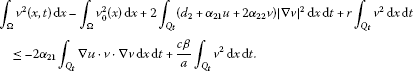(3.4)

Estimating the first term on the right-hand side of (3.4),

$\begin{array}{rcl}|{\int }_{{Q}_{t}}\mathrm{\nabla }u\cdot v\cdot \mathrm{\nabla }v\phantom{\rule{0.2em}{0ex}}\mathrm{d}x\phantom{\rule{0.2em}{0ex}}\mathrm{d}t|& =& |{\int }_{{Q}_{t}}\mathrm{\nabla }u\cdot {v}^{\frac{1}{2}}\cdot \mathrm{\nabla }v\cdot {v}^{\frac{1}{2}}\phantom{\rule{0.2em}{0ex}}\mathrm{d}x\phantom{\rule{0.2em}{0ex}}\mathrm{d}t|\\ \le & {\epsilon }_{1}{\int }_{{Q}_{t}}v{|\mathrm{\nabla }u|}^{2}\phantom{\rule{0.2em}{0ex}}\mathrm{d}x\phantom{\rule{0.2em}{0ex}}\mathrm{d}t+\frac{1}{4{\epsilon }_{1}}{\int }_{{Q}_{t}}v\cdot {|\mathrm{\nabla }v|}^{2}\phantom{\rule{0.2em}{0ex}}\mathrm{d}x\phantom{\rule{0.2em}{0ex}}\mathrm{d}t\\ \le & {\epsilon }_{2}{\int }_{{Q}_{t}}{v}^{2}\phantom{\rule{0.2em}{0ex}}\mathrm{d}x\phantom{\rule{0.2em}{0ex}}\mathrm{d}t+\frac{1}{4{\epsilon }_{2}}{\int }_{{Q}_{t}}{|\mathrm{\nabla }u|}^{4}\phantom{\rule{0.2em}{0ex}}\mathrm{d}x\phantom{\rule{0.2em}{0ex}}\mathrm{d}t\\ +\frac{1}{4{\epsilon }_{1}}{\int }_{{Q}_{t}}v\cdot {|\mathrm{\nabla }v|}^{2}\phantom{\rule{0.2em}{0ex}}\mathrm{d}x\phantom{\rule{0.2em}{0ex}}\mathrm{d}t.\end{array}$
(3.5)

Substituting (3.5) into (3.4) yields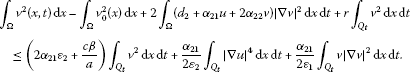(3.6)

Select ${\epsilon }_{1}>\frac{{\alpha }_{21}}{8{\alpha }_{22}}$ and denote ${C}_{4}=4{\alpha }_{22}-\frac{{\alpha }_{21}}{2{\epsilon }_{1}}$. Notice the positive equilibrium point of (1.1) exists under condition $c\beta >ar$, then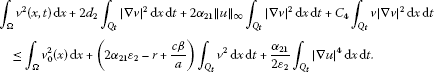(3.7)

By Lemma 2.5, ${\parallel \mathrm{\nabla }u\parallel }_{{L}^{4}}\left({Q}_{T}\right)\le {C}_{3}\left(T\right)$. Integrating the above inequality and using the Gronwall inequality, we get

$\underset{0

Hence, there exists a positive constant ${M}_{3}^{\prime }$ such that ${\int }_{\mathrm{\Omega }}{v}^{2}\phantom{\rule{0.2em}{0ex}}\mathrm{d}x\le {M}_{3}^{\prime }$. Furthermore, we have

${\parallel v\parallel }_{{L}^{2}\left({Q}_{T}\right)}\le {\int }_{0}^{T}{M}_{2}^{\prime }\phantom{\rule{0.2em}{0ex}}\mathrm{d}t\triangleq {M}_{3}.$
(3.8)

Secondly, multiplying both sides of the second equation of system (1.1) by $q{v}^{q-1}$ ($q>1$) and integrating over Ω, we have

$\begin{array}{rcl}\frac{d}{dt}{\int }_{\mathrm{\Omega }}{v}^{q}\phantom{\rule{0.2em}{0ex}}\mathrm{d}x& \le & -\frac{4\left(q-1\right){d}_{2}}{q}{\int }_{\mathrm{\Omega }}{|\mathrm{\nabla }\left({v}^{\frac{q}{2}}\right)|}^{2}\phantom{\rule{0.2em}{0ex}}\mathrm{d}x-\frac{8q\left(q-1\right){\alpha }_{22}}{{\left(q+1\right)}^{2}}{\int }_{\mathrm{\Omega }}{|\mathrm{\nabla }\left({v}^{\frac{q+1}{2}}\right)|}^{2}\phantom{\rule{0.2em}{0ex}}\mathrm{d}x\\ -q\left(q-1\right){\alpha }_{21}{\int }_{\mathrm{\Omega }}{v}^{q-1}\mathrm{\nabla }u\cdot \mathrm{\nabla }v\phantom{\rule{0.2em}{0ex}}\mathrm{d}x+q{\int }_{\mathrm{\Omega }}{v}^{q}\left(-r+\frac{c\beta mu}{1+amu}\right)\phantom{\rule{0.2em}{0ex}}\mathrm{d}x.\end{array}$

Integrating the above equation over $\left[0,t\right]$ ($t\le T$), it is clear that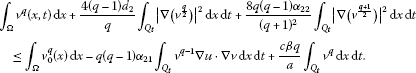(3.9)

By Lemma 2.2, it can be found that $\mathrm{\nabla }u\in {L}^{\frac{2\left(n+2\right)}{n}}\left({Q}_{T}\right)$. According to the Hölder inequality and Young inequality, we get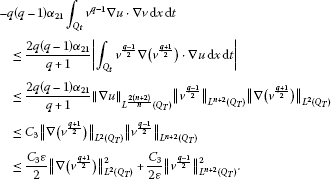(3.10)

Choose an appropriate number ε satisfying $\frac{{C}_{3}\epsilon }{2}\le \frac{8q\left(q-1\right){\alpha }_{22}}{{\left(q+1\right)}^{2}}$. Substituting (3.10) into (3.9) and taking $\overline{v}={v}^{\frac{q+1}{2}}$, we have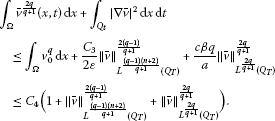(3.11)

Let

$E\equiv \underset{0

From (3.11), we know

$E\le {C}_{4}\left(1+{\parallel \overline{v}\parallel }_{{L}^{\frac{\left(q-1\right)\left(n+2\right)}{q+1}}\left({Q}_{T}\right)}^{\frac{2\left(q-1\right)}{q+1}}+{\parallel \overline{v}\parallel }_{{L}^{\frac{2q}{q+1}}\left({Q}_{T}\right)}^{\frac{2q}{q+1}}\right).$

When $q<\frac{n\left(n+4\right)}{{n}^{2}-4}$, it is easy to find that $\frac{2q}{q+1}<2<\stackrel{˜}{q}$ and $\frac{\left(q-1\right)\left(n+2\right)}{q+1}<\stackrel{˜}{q}=2+\frac{4q}{n\left(q+1\right)}$. So,

$E\le {C}_{5}\left(1+{\parallel \overline{v}\parallel }_{{L}^{\stackrel{˜}{q}}\left({Q}_{T}\right)}^{\frac{2\left(q-1\right)}{q+1}}+{\parallel \overline{v}\parallel }_{{L}^{\stackrel{˜}{q}}\left({Q}_{T}\right)}^{\frac{2q}{q+1}}\right).$
(3.12)

Set $\beta =\frac{2}{q+1}\in \left(0,1\right)$. It follows from the ${L}^{1}\left(\mathrm{\Omega }\right)$-estimate for v that

${\parallel \overline{v}\parallel }_{{L}^{\beta }\left(\mathrm{\Omega }\right)}={\left({\int }_{\mathrm{\Omega }}{|\overline{v}\left(\cdot ,t\right)|}^{\beta }\phantom{\rule{0.2em}{0ex}}\mathrm{d}x\right)}^{\frac{1}{\beta }}={\parallel v\parallel }_{{L}^{1}\left(\mathrm{\Omega }\right)}^{\frac{1}{\beta }}\le {M}_{2}^{\mathrm{\prime }\frac{1}{\beta }},\phantom{\rule{1em}{0ex}}\mathrm{\forall }t\le T.$

By Lemma 2.4 and (3.12), we know

$\begin{array}{rcl}E& \le & {C}_{5}\left[1+{\left({M}^{\prime }+{M}^{\prime }\underset{0
(3.13)

Obviously, $\frac{4\left(q-1\right)}{n\left(q+1\right)\stackrel{˜}{q}}+\frac{2\left(q-1\right)}{\left(q+1\right)\stackrel{˜}{q}}\in \left(0,1\right)$ and $\frac{4q}{n\left(q+1\right)\stackrel{˜}{q}}+\frac{2q}{\left(q+1\right)\stackrel{˜}{q}}\in \left(0,1\right)$. It is easy to know that E is bounded by use of reduction to absurdity. Since $q<\frac{n\left(n+4\right)}{{n}^{2}-4}$, $\frac{\left(q+1\right)\stackrel{˜}{q}}{2}\in \left(1,\frac{2\left(n+1\right)}{n-2}\right)$. So, ${\parallel \overline{v}\parallel }_{{L}^{\stackrel{˜}{q}}\left({Q}_{T}\right)}$ is bounded, i.e., $v\in {L}^{\frac{\left(q+1\right)\stackrel{˜}{q}}{2}}\left({Q}_{T}\right)$. Denote $\frac{\left(q+1\right)\stackrel{˜}{q}}{2}$ still as q. So,

$v\in {L}^{q}\left({Q}_{T}\right),\phantom{\rule{1em}{0ex}}\mathrm{\forall }q\in \left(1,\frac{2\left(n+1\right)}{n-2}\right).$
(3.14)

Finally, when $n=2,3,4,5$, $\left({n}^{2}-4\right)q<{n}^{2}+4n$ with $q=2$. For $n\le 5$, taking $q=2$ in (3.9), it follows from (3.8) that there exists a positive constant ${M}_{4}$ such that

${\parallel v\parallel }_{{V}_{2}\left({Q}_{T}\right)}\le {M}_{4}.$
(3.15)

By embedding theorem, we get

${\parallel v\parallel }_{{L}^{\frac{2\left(n+2\right)}{n}}\left({Q}_{T}\right)}\le {M}_{4}.$
1. (ii)

${L}^{\mathrm{\infty }}$-estimate for v.

The second equation of system (1.1) can be written as the following divergence form:

$\frac{\partial v}{\partial t}=\sum _{i,j=1}^{n}\frac{\partial }{\partial {x}_{i}}\left({a}_{ij}\left(x,t\right)\frac{\partial v}{\partial {x}_{j}}\right)+\sum _{i=1}^{n}\frac{\partial }{\partial {x}_{i}}\left({a}_{i}\left(x,t\right)v\right)+v\left(-r+\frac{c\beta mu}{1+amu}\right),$
(3.16)

where ${a}_{ij}\left(x,t\right)=\left({d}_{2}+{\alpha }_{21}u+2{\alpha }_{22}v\right){\delta }_{ij}$, ${a}_{i}\left(x,t\right)={\alpha }_{21}{u}_{{x}_{j}}$ and ${\delta }_{ij}$ is the Kronecker sign.

In order to apply the maximum principle  to (3.16), we need to prove the following conditions:

1. (1)

${\parallel v\parallel }_{{V}_{2}\left({Q}_{T}\right)}$ is bounded;

2. (2)

${\sum }_{i,j=1}^{n}{a}_{ij}\left(x,t\right){\xi }_{i}{\xi }_{j}\ge \nu {\sum }_{i=1}^{n}{\xi }_{i}^{2}$;

3. (3)

${\parallel {\sum }_{i=1}^{n}{a}_{i}^{2}\left(x,t\right),v\left(-r+\frac{c\beta mu}{1+amu}\right)\parallel }_{{L}^{p,r}\left({Q}_{T}\right)}\le {\mu }_{1}$,

where ν, ${\mu }_{1}$ are positive constants and

$\frac{1}{r}+\frac{n}{2p}=1-\chi ,\phantom{\rule{1em}{0ex}}0<\chi <1,p\in \left[\frac{n}{2\left(1-\chi \right)},+\mathrm{\infty }\right),r\in \left[\frac{1}{1-\chi },+\mathrm{\infty }\right),n\ge 2.$
(3.17)

Next, we will show that the above conditions (1) to (3) are satisfied for (3.16). When $n\le 5$, it is easy to find that the condition (1) is satisfied by use of (3.15). Since ${\sum }_{i,j=1}^{n}{a}_{ij}\left(x,t\right){\xi }_{i}{\xi }_{j}\ge {d}_{3}{|\xi |}^{2}$ for all $\xi \in {\mathbb{R}}^{n}$, the condition (2) is verified. In view of the condition (3), we take appropriate q and r. Rewrite the first equation of system (1.1) as

${u}_{t}=\mathrm{\nabla }\cdot \left[\left({d}_{1}+2{\alpha }_{11}u\right)\mathrm{\nabla }u\right]+\alpha u\left(1-\frac{u}{K}\right)-\frac{\beta muv}{1+amu}.$
(3.18)

When $n=2,3,4,5$, $\frac{n+2}{2}<\frac{2\left(n+1\right)}{n-2}$, it is clear that ${d}_{1}+2{\alpha }_{11}u$ has an upper bound over ${\overline{Q}}_{T}$ by Lemma 2.1. Set

$q\in \left(\frac{n+2}{2},\frac{2\left(n+1\right)}{n-2}\right).$

From (3.14), we have $\alpha u\left(1-\frac{u}{K}\right)-\frac{\beta muv}{1+amu}\in {L}^{q}\left({Q}_{T}\right)$. Therefore, all conditions of the Hölder continuity theorem [, Theorem 10.1] hold for (3.18). Hence,

$u\in {C}^{\beta ,\frac{\beta }{2}}\left({\overline{Q}}_{T}\right),\phantom{\rule{1em}{0ex}}\beta \in \left(0,1\right).$
(3.19)

We will discuss (2.5) which is the corresponding form of (3.18). It follows from (2.1) and (3.14) that ${C}_{1}-{C}_{2}v\in {L}^{q}\left({Q}_{T}\right)$, $\mathrm{\forall }q\in \left(\frac{n+2}{2},\frac{2\left(n+1\right)}{n-2}\right)$. From (3.19), we obtain ${d}_{1}+{\alpha }_{11}u\in {C}^{\beta ,\frac{\beta }{2}}\left({\overline{Q}}_{T}\right)$. Thus, according to the parabolic regularity result of [, pp.341-342, Theorem 9.1], we can conclude that

$X\in {W}_{q}^{2,1}\left({Q}_{T}\right),\phantom{\rule{1em}{0ex}}\mathrm{\forall }q\in \left(\frac{n+2}{2},\frac{2\left(n+1\right)}{n-2}\right),$
(3.20)

which implies that $\mathrm{\nabla }X\in {L}^{\frac{\left(n+2\right)q}{n+2-q}}\left({Q}_{T}\right)$ by Lemma 2.6.

Since $X=\left({d}_{1}+2{\alpha }_{11}u\right)u$, we have $\mathrm{\nabla }u={\left({d}_{1}+2{\alpha }_{11}u\right)}^{-1}\mathrm{\nabla }X$, i.e., $\mathrm{\nabla }u\in {L}^{\frac{\left(n+2\right)q}{n+2-q}}\left({Q}_{T}\right)$. It means that ${|\mathrm{\nabla }u|}^{2},{|\mathrm{\nabla }v|}^{2}\in {L}^{\frac{\left(n+2\right)q}{2\left(n+2-q\right)}}\left({Q}_{T}\right)$. So, ${\sum }_{i=1}^{n}{a}_{i}^{2}\left(x,t\right)\in {L}^{\frac{\left(n+2\right)q}{2\left(n+2-q\right)}}\left({Q}_{T}\right)$. From (2.1) and (3.14), $v\left(-r+\frac{c\beta mu}{1+amu}\right)\in {L}^{q}\left({Q}_{T}\right)$.

Then the condition (3) and (3.17) are satisfied by choosing $p=r=\frac{\left(n+2\right)p}{2\left(n+2-p\right)}$. According to the maximum principle [, p.181, Theorem 7.1], we can conclude that $v\in {L}^{\mathrm{\infty }}\left({Q}_{T}\right)$. From (2.1), there exists a positive constant ${M}_{5}$ such that

${\parallel u\parallel }_{{L}^{\mathrm{\infty }}\left({Q}_{T}\right)},{\parallel v\parallel }_{{L}^{\mathrm{\infty }}\left({Q}_{T}\right)}\le {M}_{5},\phantom{\rule{1em}{0ex}}\mathrm{\forall }T>0.$
(3.21)

Therefore, the global solution to the problem (1.1) exists.

1. (iii)

The existence of classical solutions.

Under the conditions of Theorem 3.1, we consider above global solutions $\left(u,v\right)$ to be classical. By (3.20) and Lemma 2.6, we know $\mathrm{\nabla }X\in {C}^{\alpha ,\frac{\alpha }{2}}\left({\overline{Q}}_{T}\right)$, $\mathrm{\forall }\alpha \in \left(0,1\right)$. It follows from Lemma 3.3 in  that $X\in {C}^{1+\alpha ,\frac{1+\alpha }{2}}\left({\overline{Q}}_{T}\right)$. Since $X=\left({d}_{1}+{\alpha }_{11}u\right)u$, we have $u=\frac{-{d}_{1}\sqrt{{d}_{1}^{2}+4{\alpha }_{11}X}}{2{\alpha }_{11}}$.

So,

$u\in {C}^{1+\alpha ,\frac{1+\alpha }{2}}\left({\overline{Q}}_{T}\right),\phantom{\rule{1em}{0ex}}\mathrm{\forall }\alpha \in \left(0,1\right).$
(3.22)

Rewrite the second equation of system (1.1) as

${v}_{t}=\mathrm{\nabla }\cdot \left[\left({d}_{2}+{\alpha }_{21}u+2{\alpha }_{22}v\right)\mathrm{\nabla }v+{\alpha }_{21}\mathrm{\nabla }uv\right]+v\left(-r+\frac{c\beta mu}{1+amu}\right).$

Therefore, we can conclude that $v\left(-r+\frac{c\beta mu}{1+amu}\right)\in {L}^{\mathrm{\infty }}\left({Q}_{T}\right)$, u, v, u and v are all bounded. By the Schauder estimate , there exists ${\alpha }^{\ast }\in \left(0,1\right)$ such that

$v\in {C}^{{\alpha }^{\ast },\frac{{\alpha }^{\ast }}{2}}\left({\overline{Q}}_{T}\right).$
(3.23)

Furthermore, by the Schauder estimate, we obtain

$u\in {C}^{2+\sigma ,1+\frac{\sigma }{2}}\left({\overline{Q}}_{T}\right),\phantom{\rule{1em}{0ex}}\sigma =min\left\{\alpha ,{\alpha }^{\ast }\right\}.$
(3.24)

Next, the regularity of v will be discussed. Set $\overline{v}=\left({d}_{2}+{\alpha }_{21}u+{\alpha }_{22}v\right)v$. So, $\overline{v}$ satisfies

${\overline{v}}_{t}=\left({d}_{2}+{\alpha }_{21}u+2{\alpha }_{22}v\right)\mathrm{\Delta }\overline{v}+f\left(x,t\right),$
(3.25)

where $f\left(x,t\right)=\left({d}_{2}+{\alpha }_{21}u+2{\alpha }_{22}v\right)v\left(-r+\frac{c\beta mu}{1+amu}\right)+{\alpha }_{21}{u}_{t}v$. According to (3.22) to (3.24), we have ${d}_{2}+{\alpha }_{21}u+2{\alpha }_{22}v$, $f\left(x,t\right)\in {C}^{\sigma ,\frac{\sigma }{2}}\left({\overline{Q}}_{T}\right)$. Applying the Schauder estimate to (3.25), we know

$\overline{v}\in {C}^{2+\sigma ,1+\frac{\sigma }{2}}\left({\overline{Q}}_{T}\right).$

From $v=\frac{-\left({d}_{3}+{\alpha }_{21}u\right)+\sqrt{{\left({d}_{2}+{\alpha }_{21}u\right)}^{2}+4{\alpha }_{22}\overline{v}}}{2{\alpha }_{22}}$, we can see

$v\in {C}^{2+\sigma ,1+\frac{\sigma }{2}}\left({\overline{Q}}_{T}\right),\phantom{\rule{1em}{0ex}}\sigma =min\left\{\alpha ,{\alpha }^{\ast }\right\}.$
(3.26)

Combining (3.24) and (3.26), we get

$u,v\in {C}^{2+\sigma ,1+\frac{\sigma }{2}}\left({\overline{Q}}_{T}\right).$

Therefore, the result of Theorem 3.1 can be obtained for $\alpha <{\alpha }^{\ast }$, namely $\sigma =\alpha$. When $\alpha >{\alpha }^{\ast }$, namely $\sigma <\alpha$, we have ${C}^{2+\sigma ,1+\frac{\sigma }{2}}\left({\overline{Q}}_{T}\right)↪{C}^{\alpha ,\frac{\alpha }{2}}\left({\overline{Q}}_{T}\right)$. (3.24) and (3.26) are obtained by repeating the above bootstrap argument and the Schauder estimate. This completes the proof of Theorem 3.1. □

## References

1. Cao H, Fu S: Global existence and convergence of solutions to a cross-diffusion cubic predator-prey system with stage structure for the prey. Bound. Value Probl. 2010. doi:10.1155/2010/285961

2. Dubey B, Das B, Hussain J: A predator-prey interaction model with self and cross-diffusion. Ecol. Model. 2001, 141: 67-76. 10.1016/S0304-3800(01)00255-1

3. Ko W, Ryu K: Qualitative analysis of a predator-prey model with Holling type II. Functional response incorporating a prey refuge. J. Differ. Equ. 2006, 231(2):534-550. 10.1016/j.jde.2006.08.001

4. Amann H: Dynamic theory of quasilinear parabolic equations - I. Abstract evolution equations. Nonlinear Anal. 1988, 12: 859-919.

5. Amann H: Dynamic theory of quasilinear parabolic equations - II. Reaction-diffusion. Differ. Integral Equ. 1990, 3: 13-75.

6. Amann H: Dynamic theory of quasilinear parabolic equations - III. Global existence. Math. Z. 1989, 202: 219-250. 10.1007/BF01215256

7. Lou Y, Ni Y, Wu Y: On the global existence of a cross-diffusion system. Discrete Contin. Dyn. Syst. 1998, 4: 193-203.

8. Tuoc, PV: On global existence of solutions to a cross-diffusion system. IMA Preprint Series 2149

9. Choi YS, Lui R, Yamada Y: Existence of global solutions for the Shigesada-Kawasaki-Teramoto model with strongly coupled cross-diffusion. Discrete Contin. Dyn. Syst. 2004, 10(3):719-730.

10. Shim S: Uniform boundedness and convergence of solutions to cross-diffusion systems. J. Differ. Equ. 2002, 185: 281-305. 10.1006/jdeq.2002.4169

11. Shim S: Uniform boundedness and convergence of solutions to the systems with cross-diffusion dominated by self-diffusion. Nonlinear Anal., Real World Appl. 2003, 4: 65-86. 10.1016/S1468-1218(02)00014-7

12. Shim S: Uniform boundedness and convergence of solutions to the systems with a single nonzero cross-diffusion. J. Math. Anal. Appl. 2003, 279: 1-21. 10.1016/S0022-247X(03)00045-3

13. Ladyzenskaja OA, Solonnikov VA, Uralceva NN Translations of Mathematical Monographs 23. In Linear and Quasilinear Equations of Parabolic Type. Am. Math. Soc., Providence; 1968.

## Acknowledgements

The author thanks the anonymous referee for a careful review and constructive comments. This work was supported by the Qinghai Provincial Natural Science Foundation (2011-Z-915).

## Author information

Authors

### Corresponding author

Correspondence to Yanzhong Zhao.

### Competing interests

The author declares that he has no competing interests.

### Authors’ contributions

The work was realized by the author.

## Rights and permissions

Reprints and Permissions

Zhao, Y. Global behavior for a diffusive predator-prey system with Holling type II functional response. Bound Value Probl 2012, 111 (2012). https://doi.org/10.1186/1687-2770-2012-111